Technical Information Site of Power Supply Design

2021.07.14 Simulation

# PFC Circuits: Adjustment of Switching Frequency

Optimization of PFC Circuits

PFC Circuits: Adjustment of Switching Frequency

Previously, a method in which the inductance of an inductor is adjusted was presented as a method of correcting the ripple ratio of an inductor current after modifications to circuit conditions. As a separate method, here we introduce a technique in which the switching frequency fSW is adjusted. The operating mode is assumed to be CCM (continuous current mode).

Example Circuit

As an example circuit, the simulation circuit "A-6. PFC CCM Synchro Vin=200V Iin=2.5A" in the Power Device Solution Circuit/AC-DC PFC list is used (see Fig. 6). Details of the circuit diagram can also be seen here.

In this example, when the conditions within the yellow box in Fig. 6 are changed, the switching frequency is adjusted so as to optimize the ripple ratio of the inductor current. The result is confirmed through a simulation.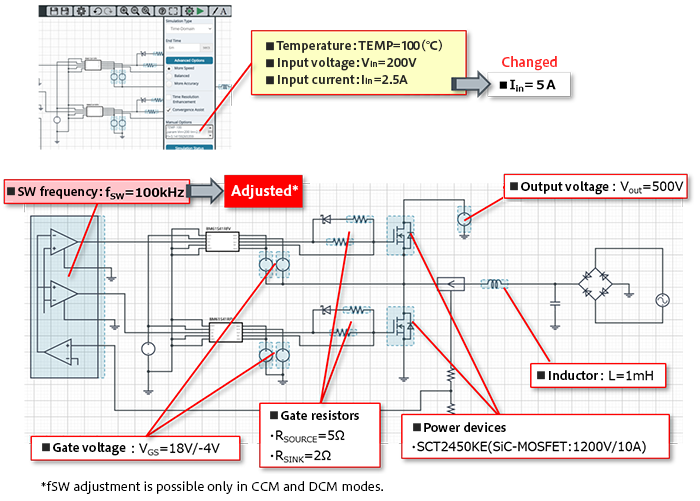Fig. 6: The "A-6. PFC CCM Synchro Vin=200V Iin=2.5A" PFC simulation circuit, and modified conditions

Ripple Ratio Before Switching Frequency Adjustment

Fig. 7 shows the inductor current IL before adjustment of the switching frequency fSW (default value: 100 kHz). The peak value is IL_peak ≈ 7.8 A.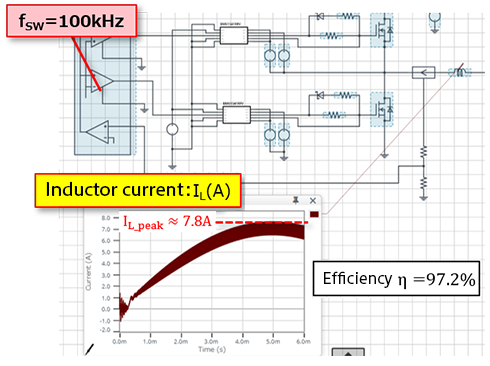Fig.7: Inductor current IL prior to adjustment of the switching frequency fSW (default value: 100 kHz)

The input current peak value is Iin_peak=√2×Iin ≈ 7.07 A, and so from these values the ripple ratio M is calculated as follows.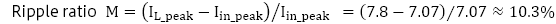This is sufficiently smaller than what is widely regarded as sufficient, a ripple ratio value of under 30%, and so the possibility of lowering fSW and modifying the ripple ratio to improve efficiency is studied. The efficiency prior to adjustment of fSW is η = 97.2%.

The switching frequency fSW is generally calculated as follows.For η = 0.972 and the generally used value for the ripple ratio of 30% (0.3), fSW is calculated as follows.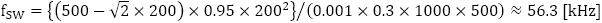When the switching frequency is changed to fSW = 55 kHz based on the calculation results and a simulation is again run, the inductor current peak value is IL_peak ≈ 8.5 A, as shown in Fig. 8. The ripple ratio in this case is M = (8.5-7.07)/7.07 ≈ 20.2%. The efficiency is η = 97.3%, an improvement of 0.1% from before fSW adjustment. Through this adjustment of fSW, we see that it is possible to improve efficiency while maintaining an appropriate inductor current ripple ratio.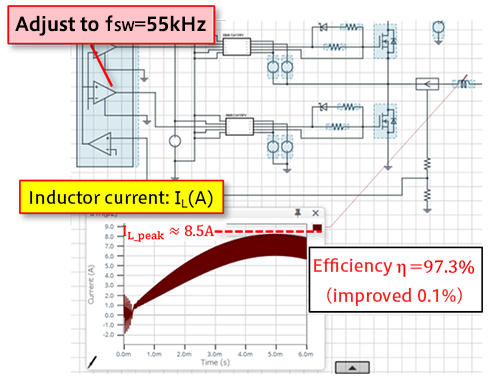Figure 8: Inductor current IL when the switching frequency fSW is adjusted to 55 kHz

#### Key Points:

・An example is given in which, when operating conditions are changed in a PFC Synchronous CCM circuit, the possibility of improving efficiency while maintaining an appropriate inductor current ripple ratio by adjusting the switching frequency is studied.

・A switching frequency resulting in an appropriate ripple ratio is calculated, and a simulation is performed to verify the result.